# Price is what you pay

Stock analysts are usually separated into two philosophical camps: fundamental or technical. The fundamental analyst uses financial statements, economic forecasts, industry knowledge, and valuation to guide his or her investment process. The technical analyst uses prices, charts, and a whole host of “indicators”. In reality, few stock analysts are purely fundamental or technical, usually blending a combination of the tools based on temperament, experience, and past success. Nonetheless, at the end of the day, the fundamental analyst remains most concerned with valuation, while the technical focuses on price action.

But what is valuation? Generally, it is how much a company is worth. But how does one calculate how much it’s worth? One way is to generate forecasts of a business’s profitability and use various tools to estimate the riskiness of the business and exposure to macroeconomic factors to arrive at some number that is said to be the (highly approximate!) value of the company today. Another way is to figure out what other people are have been willing to pay for it and then see if that jibes with the price today.

For this, and succeeding, posts on this topic we’ll look at the second method—figuring out what other folks are willing to pay. And we’ll rely on one of the most frequently quoted, though perhaps less understood, metrics—price-to-earnings. But before we get into that, we want to acknowledge SimFin, which is a website that provides financial statement data of public companies in an easy-to-use format. There are few sources of freely available, quality fundamental financial data and SimFin is one of the best that we’ve found. We used SimFin to compile the data in this post. We also want to acknowledge Marcelo S. Perlin, whose package simfinR (still in development), was critical in helping us compile that data efficiently. Please check out Marcelo’s high quality blog. It’s well worth the time.

Now onto the post. One way to figure out what folks are willing to pay for a stock is to look at historical multiples. The most common being price-to-earnings, which is simply the stock price divided by the earnings-per-share, hence multiple. There is a perennial debate on the validity, usefulness, and/or rigor of multiples. We won’t spend time on such debates here. But we want to flag two key points. Most investment practitioners (research analysts, portfolio managers, and M&A bankers) use or have used multiples at some point. So even if multiples are a lazy, short-hand, unintellectual, and sloppy methodology, they still represent convention. Second, some investment strategists have attempted to establish the link between the multiple and more rigorous drivers of value like discount rates and return on investment. That too, is beyond this discussion, but for those interested, check out this article.

A major reason to use historical multiples, besides the ease of calculation, is that they represent the market’s appraisal of a company’s value at a point-in-time. If the market is generally right, or efficient as economists like to say, then the multiple of earnings implied by the price should approximate the value of a particular company most of the time. Thus, if a stock is priced (or trading) at a multiple below it’s typical level, it is cheap, and if above, expensive. If you buy that stock at a cheap multiple, you’ve effectively bought a part of the underlying company at a discount. And when the stock returns to its normal multiple, you’ll have generated a tidy return; that is, if you bought it at a cheap enough multiple. Folks that look for stocks priced cheaply (often based on low multiples) are frequently called value investors, of which Warren Buffett is the most famous. Although, in reality no looks to buy overvalued stocks.

There are a whole host of problems with using multiple-based approach. Too many to enumerate completely. But, to name a few, these problems include the fact that multiples and earnings change over time; the reasons for such variability can be both systemic or idiosyncratic; there is no law that requires multiples to revert to former levels; and the consensus on the right multiple today may not be the consensus tomorrow. Additionally, there are methodological issues. Should one use historical or projected earnings. Accounting or adjusted earnings? What about companies with no earnings or depressed earnings? Many of these issues are addressable either with rigor or hand-waving. But let’s forget all that for a moment.

Assuming relatively stability, a stock’s multiple might be able tell you something about future returns based on empirical evidence. And that information might be able to be used to formulate an investing strategy. For example, if you noticed that every time stock XYZ hit 10x earnings it tended to go up and every time it surpassed 20x earnings it tended to fall, that might be an exploitable phenomenon in the future. How would one test this?

Now we get into the meat of the data science part and the power of R. True, a lot of this could be done with a spreadsheet. But it’s laborious and difficult to replicate or automate. To illustrate the mulitple-based approach will look at Apple, whose stock ticker is AAPL. We use Apple simply because it is a relatively well-known stock, not because we like or own the stock or making investment recommendations. But Apple’s shae price should also provide a good example of some of the pitfalls associated with the multiple approach. As always most of the code will run in the background, but will be reprinted below for reference.

First, we’ll need to load a bunch of packages (including simfinR), pull the data, wrangle it to get the right earnings, calculate the multiples, and then do some exploratory analysis before trying to test out any strategies. Here is the requisite price chart.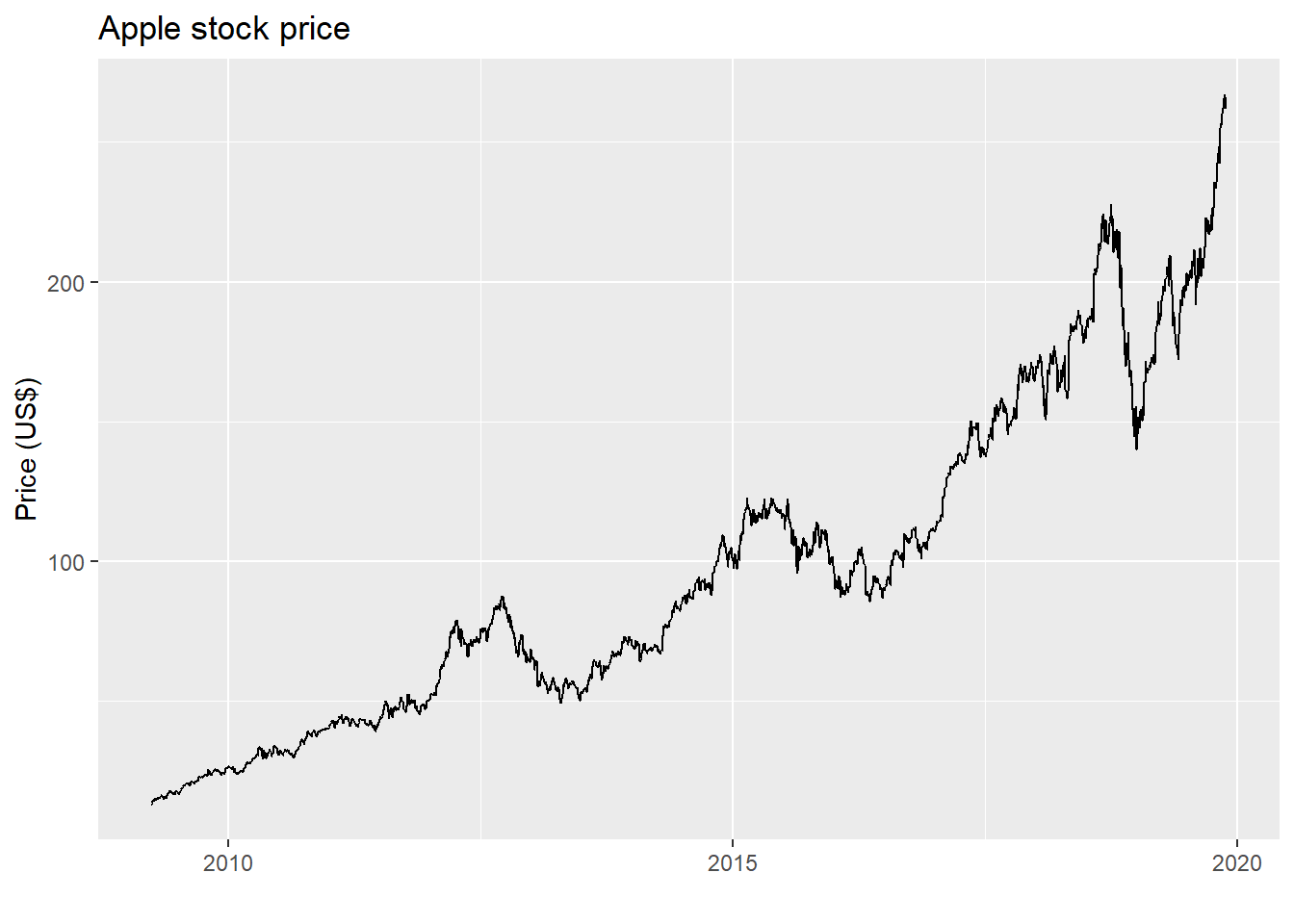And here is the graph of Apple’s price-to-earnings multiple based on its last twelve months of earnings, otherwise known as the LTM P/E. In this case, we divide the current price by the rolling four quarter sum of Apple’s earnings-per-share based on its diluted share count.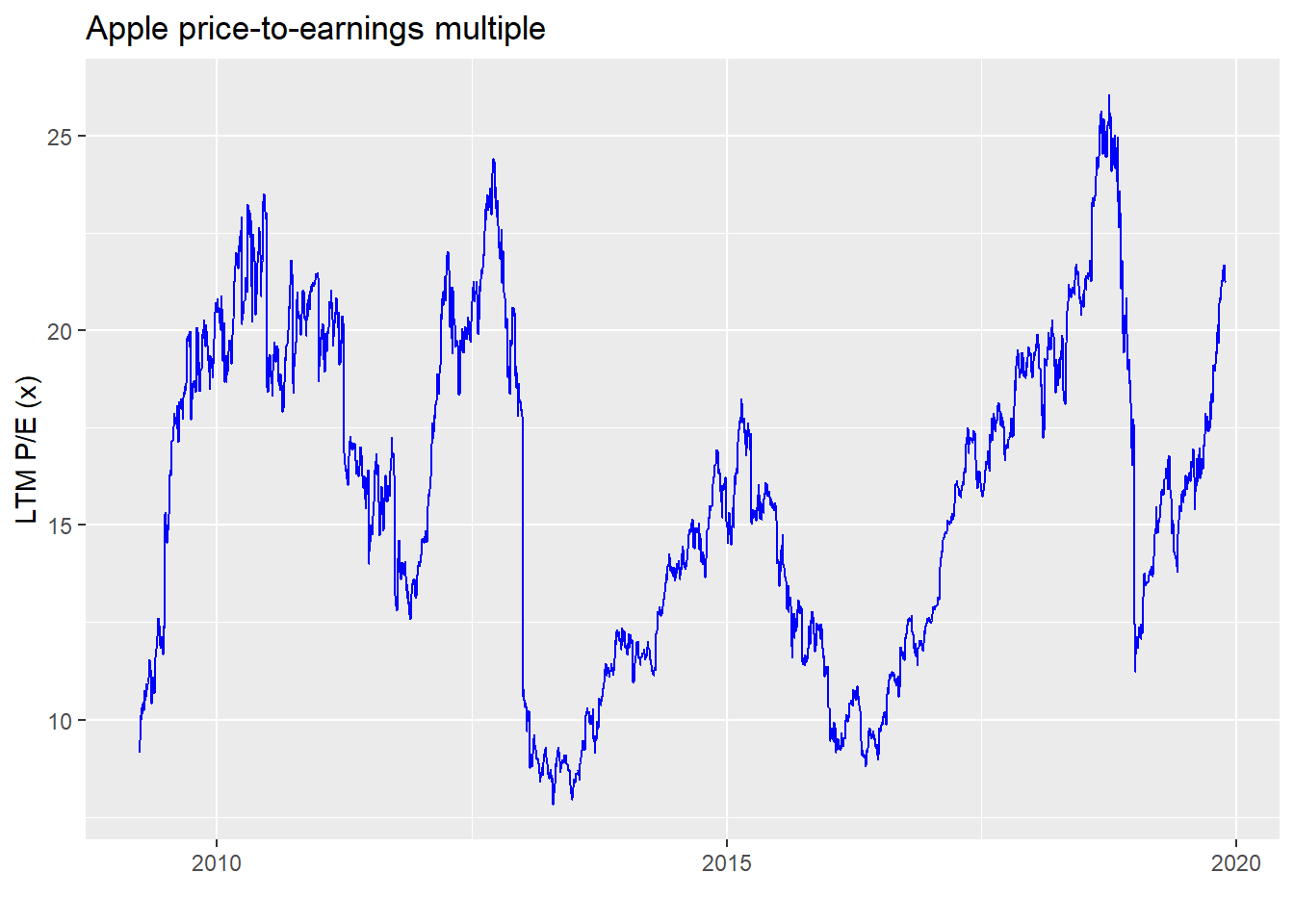Notice anything? Apple’s multiple tends to average about 16x, rarely going below 10 or above 20. Here are the summary statistics.

Table 1: Apple multiple summary statistics
Average Std. deviation Minimum Maximum
15.9 4.2 7.8 26.1

Now let’s put thoe two charts together to get a sense of how the multiple trends with the stock price.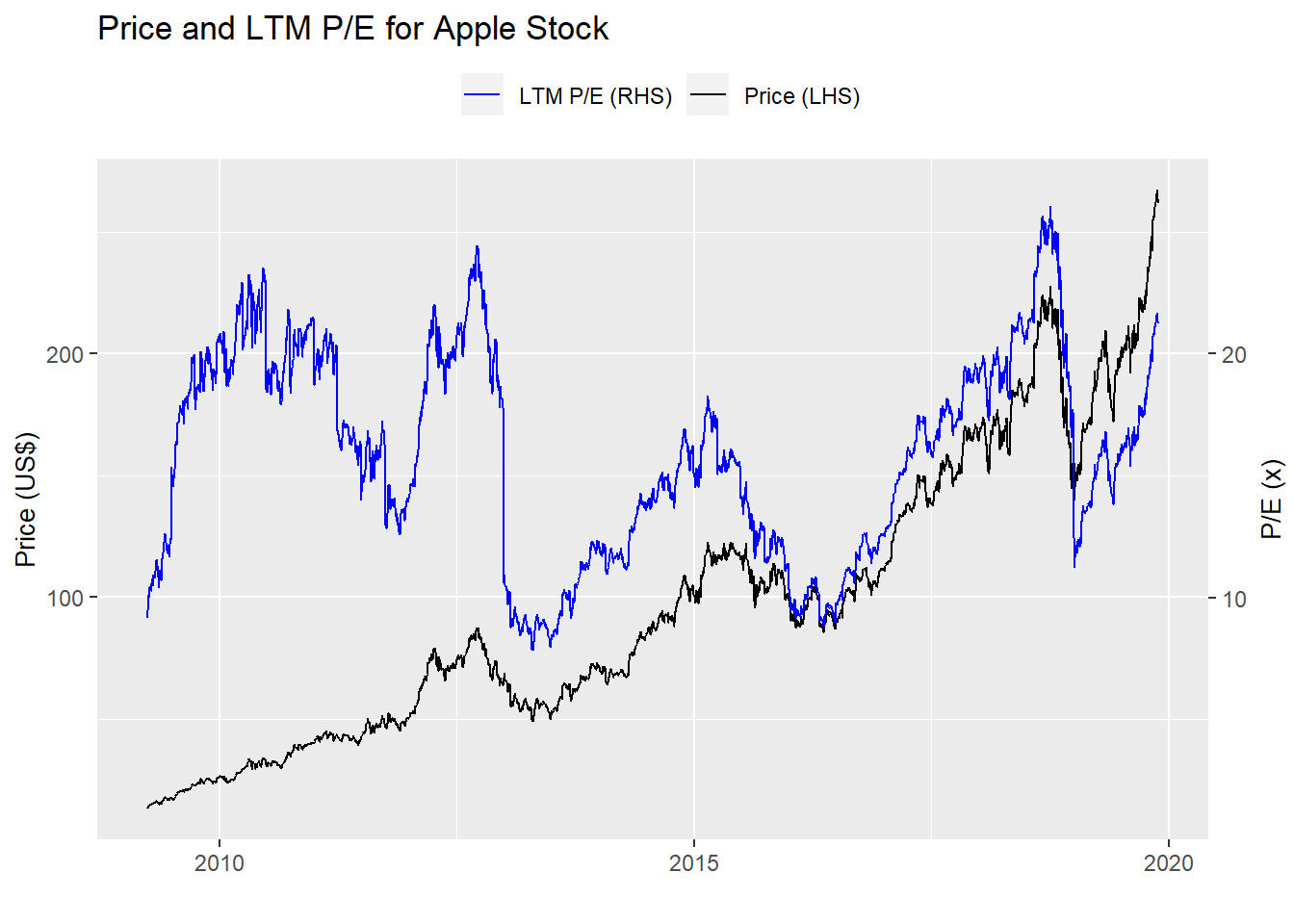Now let’s look at a histogram of Apple’s multiple to get a sense of how frequent the different levels of the multiple are across time. Here we overlay the histogram with the normal distribution.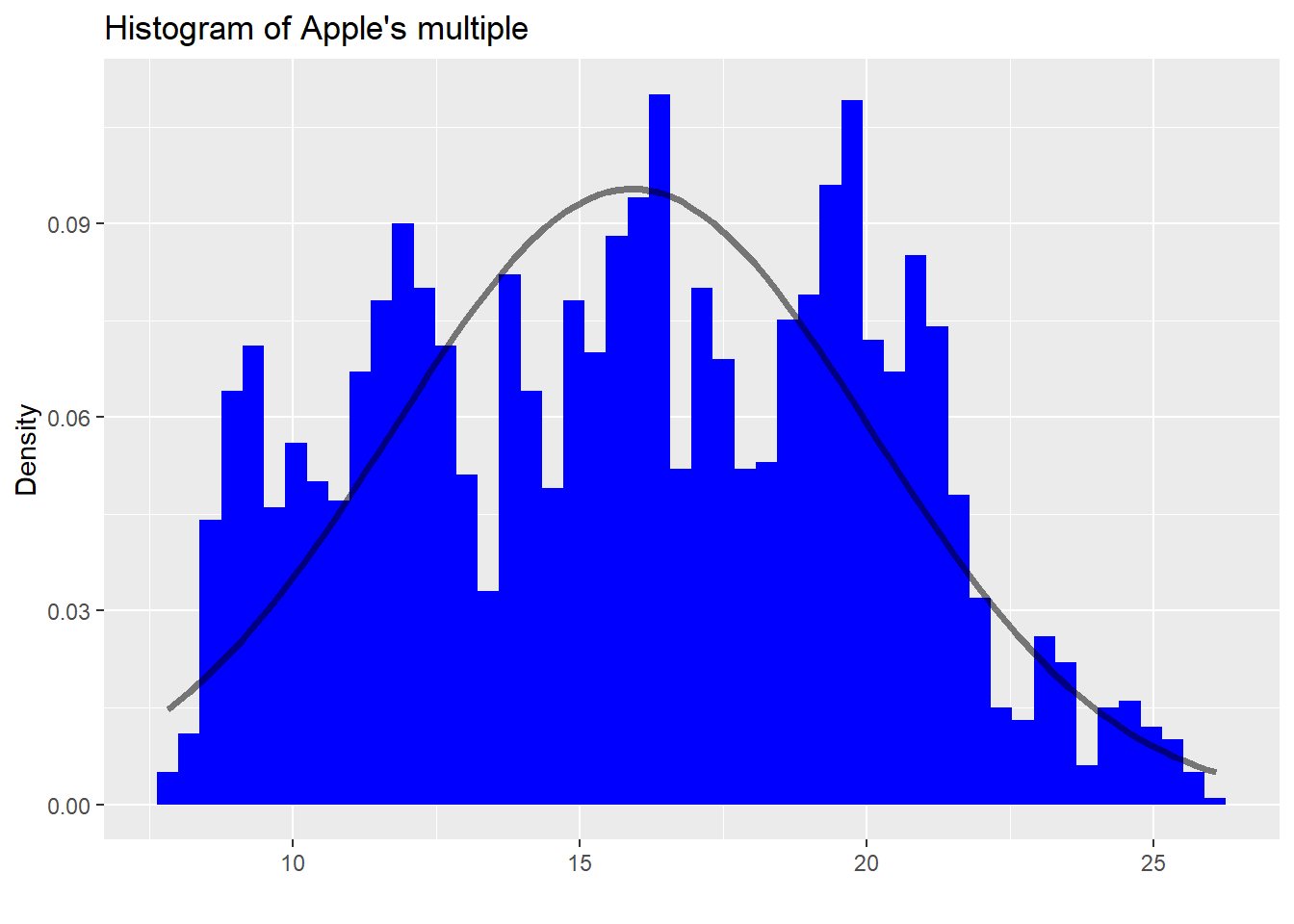What an odd-looking graph. One thing is clear: Apple’s multiple is not normally distributed. There are far more occurrences of the multiple at greater than one standard deviation than one would anticipate. Given such an odd shape to the distribution, we should already be skeptical about using standard statistical assumptions to infer future patterns. We could look at some other graphs to check autocorrelation and stationarity. But we want to build an intuition first on whether valuation has any impact on price performance. We’ll have to gloss over those aspects for now.

Moving on, we calculate the one, three, six, and twelve month returns for Apple. Then we regress those returns against the multiple. We display the results in the table below.

Table 2: Model summary outputs
Models Size effect P-value R-squared (%)
One-month -0.002 0.000 1.6
Three-month -0.009 0.000 6.5
Six-month -0.016 0.000 11.8
One-year -0.027 0.000 13.9

We see that in all cases, multiples have an inverse relationship with returns as denoted by the negative sign on the size effect. For example, for every point increase in the multiple, the one-year forward return declines by 2.7% points. That should make sense. As multiples go up, the relative cheapness of a stock goes down, until at some point, the shares no longer offer an attractive valuation. Incremental buyers dry up. Then the share price recedes. We note that the significance of the effect is quite high since all the p-values (roughly the chance that these results are no different than random occurrences) are below 5%. Finally, we see that the R-squareds (or how much the multiples explain the variability in returns) are relatively low. Even for the one-year return the R-squared is below 20%. This isn’t surprising and it supports our intuitions to a certain degree. First, there are many factors that explain the variability in stock price returns from the macro—market returns, interest rates, and GDP – to the micro – unit sales, product upgrades, and customer churn to name a few. Additionally, short-term gyrations are more likely due to supply and demand factors – one fund is rebalancing its portfolio, another wants to alter its risk exposure, etc.—that have nothing to do with the variables that drive long-term value. What is interesting and does suggest that valuation has more impact over a longer timeframe is that the R-squared does increase over time. Of course, something else could be causing that increase and we’d really need to check that the increase is significant. But that is a good first guess.

Now let’s test how well using valuation to predict stock price moves stands up to out-of-sample data. We’ll train the models up to a certain date and then test that model on the out-of-sample data. Here, we arbitrarily choose 2009-2015 as the training set, and 2016-2018 as the test set. We could get more nuanced and employ time-series cross-validation, but that’s getting ahead of ourselves. To show how well the models perform, we graph the root mean squared errors below for each return time series we examine for both the training and test sets.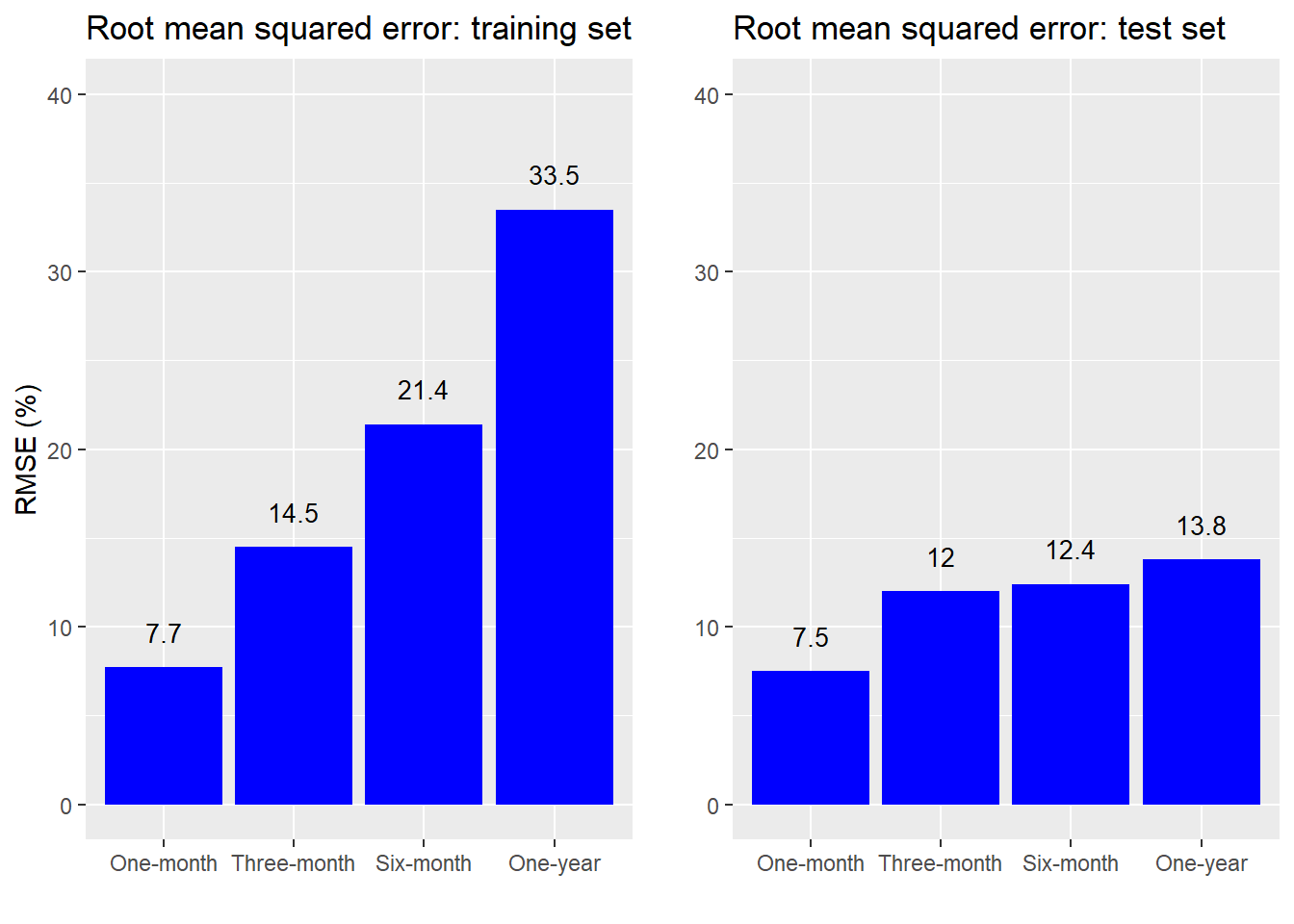Remarkable! The model actually performs better on the test sets than it does on the training set! We’re amazing forecasters. Actually, we have a very good explanation for why the out-of-sample accuracy was so good, but we’ll hold off on that second. Let’s drill down into the data a bit more.

What stands out within the individual graphs is how the forecast accuracy erodes over time. That is, the valuation today is more accurate in predicting returns over the next month than over the next year. This is intuitive for a couple of reasons. First, more things can happen in a longer timeframe than can be encapsulated by a single metric. Second, if there is even a modest bit of efficiency in the market, then multiples that are outside of the normal range should correct quickly making them better at forecasting near-term returns. As a side note, the worsening forecast accuracy for longer time periods may seem to contradict our findings that the R-squareds improved with longer periods. It doesn’t actually and that’s due to the math and the market trend.1 And it is the trend that explains the better out-of-sample forecast accuracy—the stock price and the multiple were more highly correlated during the test period. Just look at the graph of Apple’s stock price and multiple since 2016.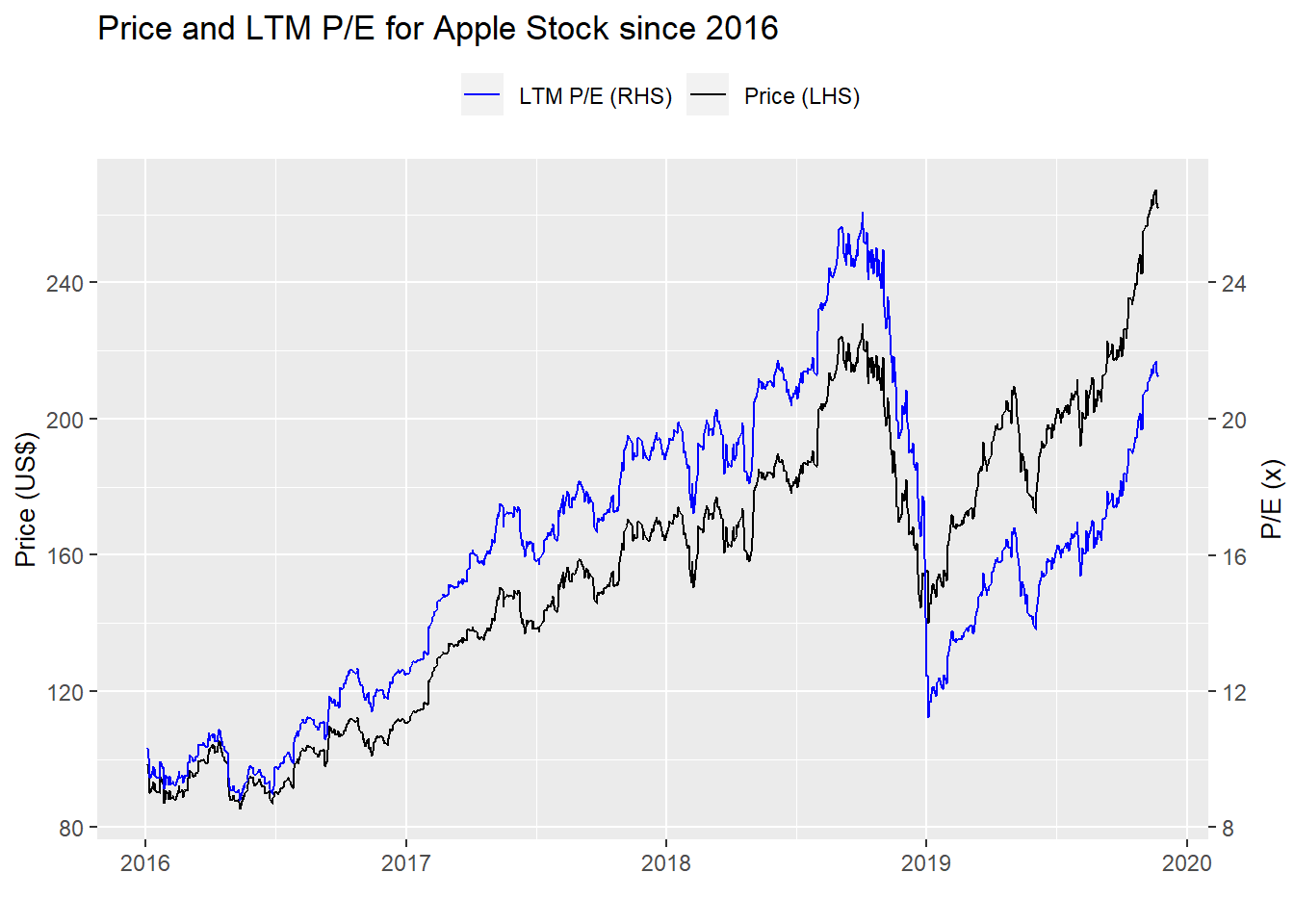This provides a good lesson on how to be a great forecaster—choose a future period in which correlations go up!

Joking aside, where do we stand? We note that the relationship between Apples’s multiple and its return is statistically significant. But the multiple has only modest explanatory power with respect to the overall variability of returns. In the short-term Apple’s multiple has better forecast accuracy than in the long term, but the sample period plays a critical factor too.

Should someone build an investing strategy around Apple’s multiple? It’s possible, but it’s not clear it would generate attractive returns. We’ll look at how one might do so in an upcoming post. For now, here is the code behind the previous analysis and graphs.

# Load package
library(tidyquant)
library(simfinR)
library(httr)
library(jsonlite)

# Set up keys
my_api_key <- "apikey" # from simfin

# Get simId
df_info_companies <- simfinR_get_available_companies(my_api_key)
df_info_companies %>%
filter(ticker == "AAPL") %>%
select(simId)
# 111052

aapl <- 111052
statements = c("pl")
periods = c('Q1', 'Q2', 'Q3', 'Q4') # final year
years = 2008:2019

aapl_pl <- simfinR_get_fin_statements(aapl,
my_api_key,
type_statements = statements,
periods = periods,
years = years)

aapl_pl %>%
filter(acc_name == "Net Income",
acc_value !=0) %>%
select(ref_date, acc_name, acc_value) %>%

url <- paste("https://simfin.com/api/v1/companies/id/111052/shares/aggregated", "?api-key=",
my_api_key,
"&type=","common",
"$measure", "period", "&ptype=", "TTM", "&fyear=", 2019, sep="") get_data <- GET(url) aapl_shares <- fromJSON(content(get_data, "text"),flatten = TRUE) # Load price data aapl_price <- simfinR_get_price_data(id_companies = aapl, api_key = my_api_key) ## Clean data # Net inocme net_income <- aapl_pl %>% filter(acc_name == "Net Income", acc_value !=0) %>% select(ref_date, acc_name, acc_value) %>% spread(acc_name, acc_value) %>% rename(date = "ref_date", net_income = "Net Income") net_income <- net_income %>% mutate(ltm_net = net_income + lag(net_income, 1) + lag(net_income, 2) + lag(net_income,3), annl_net = net_income*4) %>% mutate(ltm_net = ifelse(is.na(ltm_net), net_income*4, ltm_net)) # Diluted shares outstanding dil_shares <- aapl_shares %>% filter(figure == "common-outstanding-diluted", period %in% c("Q1", "Q2", "Q3", "Q4")) %>% select(date, figure, value) %>% spread(figure, value) %>% rename(shares = "common-outstanding-diluted") %>% mutate(date = ymd(date), shares = as.numeric(shares)) # Price price <- aapl_price %>% select(ref_date, close_adj) %>% rename(date = "ref_date", price = "close_adj") %>% arrange(date) %>% filter(date >= "2009-03-31") # Create multiple data.frame dat <- net_income %>% left_join(dil_shares, by = "date") multiple <- price %>% left_join(dat, by = "date") %>% na.locf() %>% mutate(ltm_eps = ltm_net/shares, annl_eps = annl_net/shares, pe_ltm = price/ltm_eps, pe_annl = price/annl_eps) # Price chart multiple %>% ggplot(aes(date)) + geom_line(aes(y = price), color = "blue") + labs(title = "Apple stock price", x = "", y = "Price (US$)")

# Multiple
multiple %>%
ggplot(aes(date)) +
geom_line(aes(y = pe_ltm), color = "black") +
labs(title = "Apple price-to-earnings multiple",
x = "",
y = "LTM P/E (x)")

# Multiple table
multiple %>%
summarise(Average = round(mean(pe_ltm),1),
"Std. deviation" = round(sd(pe_ltm),1),
Minimum = round(min(pe_ltm),1),
Maximum = round(max(pe_ltm),1)) %>%
knitr::kable(caption = "Apple multiple summary statistics")

# Multiple histogram with normal distribution
multiple %>%
ggplot(aes(pe_ltm)) +
geom_histogram(aes(y = ..density..),
bins = 50,
fill = "blue" ) +
stat_function(fun = dnorm,
args = list(mean = mean(multiple$pe_ltm), sd = sd(multiple$pe_ltm)),
lwd = 1.25,
alpha = 0.5) +
labs(title = "Histogram of Apple's multiple",
x = "",
y = "Density")

# Create data frame
mult_mod <- multiple %>%
ret_6m = lead(price, 132)/price - 1,
ret_12m = lead(price, 252)/price - 1)

# Run models
model_list <- list()
y_vars <- c("ret_1m", "ret_3m", "ret_6m", "ret_12m")

for(vars in y_vars){
forms <- as.formula(paste(vars, "pe_ltm", sep = " ~ "))
model_list[[vars]] <- lm(forms, mult_mod)
}

# Produce results
mod_out <- data.frame(mods = y_vars,
size_eff = rep(0,4),
p_vals = rep(0,4),
rsqs = rep(0,4))

for(i in 1:length(model_list)){
mod_out[i,2] <- as.numeric(summary(model_list[[i]])$coeff[2,1]) mod_out[i,3] <- as.numeric(summary(model_list[[i]])$coeff[2,4])
mod_out[i,4] <- as.numeric(summary(model_list[[i]])r.squared) } mod_out %>% mutate(mods = case_when(mods == "ret_1m" ~ "One-month", mods == "ret_3m" ~ "Three-month", mods == "ret_6m" ~ "Six-month", mods == "ret_12m" ~ "One-year"), size_eff = round(size_eff, 3), p_vals = format(round(p_vals,3), nsmall = 3), rsqs = round(rsqs,3)*100) %>% rename("Models" = mods, "Size effect" = size_eff, "P value" = p_vals, "R-squared (%)" = rsqs) %>% knitr::kable(caption = "Model summary outputs", align = c("l", "r", "r", "r")) # Training models train <- mult_mod %>% filter(date < "2016-01-01") test <- mult_mod %>% filter(date >= "2016-01-01", date <= "2018-12-31") # RMSE on training model train_mods <- data.frame(mods = y_vars, rmsqs = rep(0,4)) for(i in 1:length(y_vars)){ forms <- as.formula(paste(y_vars[i], "pe_ltm", sep = " ~ ")) lm_mod <- lm(forms, train) lm_pred <- predict(lm_mod, train) train_mods[i,2] <- sqrt(mean((lm_pred - train[,y_vars[i]])^2, na.rm = TRUE)) } # RMSE on tests test_mods <- data.frame(mods = y_vars, rmsqs = rep(0,4)) for(i in 1:length(y_vars)){ forms <- as.formula(paste(y_vars[i], "pe_ltm", sep = " ~ ")) lm_mod <- lm(forms, train) lm_pred <- predict(lm_mod, test) test_mods[i,2] <- sqrt(mean((lm_pred - test[,y_vars[i]])^2, na.rm = TRUE)) } # Graph train and test RMSE train_graph <- train_mods %>% mutate(mods = case_when(mods == "ret_1m" ~ "One-month", mods == "ret_3m" ~ "Three-month", mods == "ret_6m" ~ "Six-month", mods == "ret_12m" ~ "One-year"), rmsqs = round(rmsqs, 3)*100) %>% ggplot(aes(reorder(mods,rmsqs), rmsqs)) + geom_bar(stat = "identity", fill = "blue") + labs(title = "Root mean squared error for training set", x = "", y = "RMSE (%)") + scale_y_continuous(limits = c(0,40)) + geom_text(aes(y = rmsqs, label = rmsqs), nudge_y = 2, size = 3.5) test_graph <- test_mods %>% mutate(mods = case_when(mods == "ret_1m" ~ "One-month", mods == "ret_3m" ~ "Three-month", mods == "ret_6m" ~ "Six-month", mods == "ret_12m" ~ "One-year"), rmsqs = round(rmsqs, 3)*100) %>% ggplot(aes(reorder(mods,rmsqs), rmsqs)) + geom_bar(stat = "identity", fill = "blue") + labs(title = "Root mean squared error for test set", x = "", y = "") + scale_y_continuous(limits = c(0,40)) + geom_text(aes(y = rmsqs, label = rmsqs), nudge_y = 2, size = 3.5) gridExtra::grid.arrange(train_graph, test_graph, nrow = 1) # From 2016 multiple %>% filter(date >= "2016-01-01") %>% ggplot(aes(date)) + geom_line(aes(y = price, color = "Price")) + geom_line(aes(y = pe_ltm*10, color = "LTM P/E")) + scale_color_manual("", values = c("blue", "black")) + scale_y_continuous(name = "Price (US)",
sec.axis = sec_axis(~./10, name = "P/E (x)")) +
labs(title = "Price and LTM P/E for Apple Stock since 2016",
x = "") +
theme(legend.position = "top", axis.title.y.right = element_text(angle = 90, size = 10),
axis.title.y.left = element_text(size = 10))

1. Over a longer timeframe returns are likely to have wider range of outcomes meaning error rate goes up. But, even as the error rate increases, so does the deviation, often at a faster pace. This means that more of the variation appears to be “explained”. since R-squared is one minus the sum of the squared residuals divided by the total sum of squares. Additionally, in an upwardly trending market, returns will be higher for longer timeframes, than shorter ones (all things being equal), so the average return should be higher. And if the gradient of the trend is moderate, the deviation from the average will be modest too.